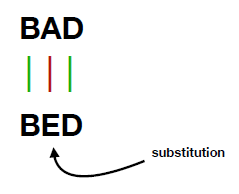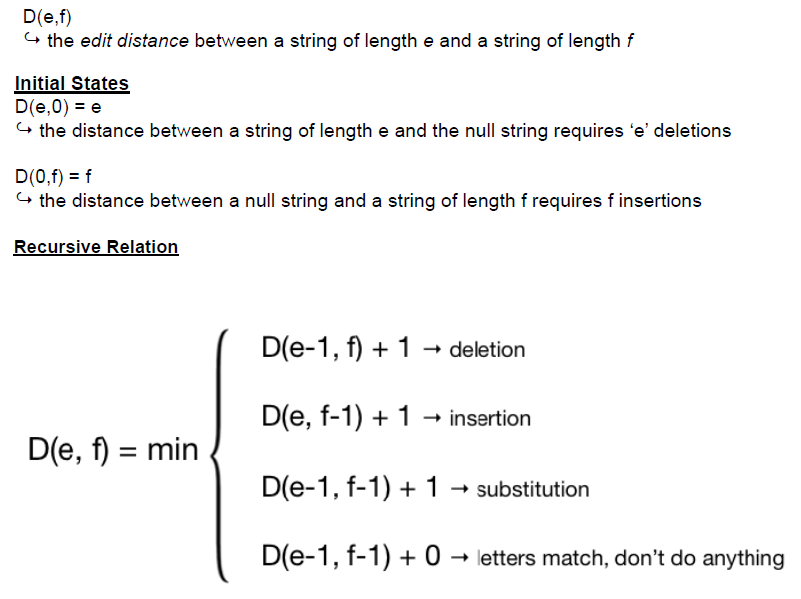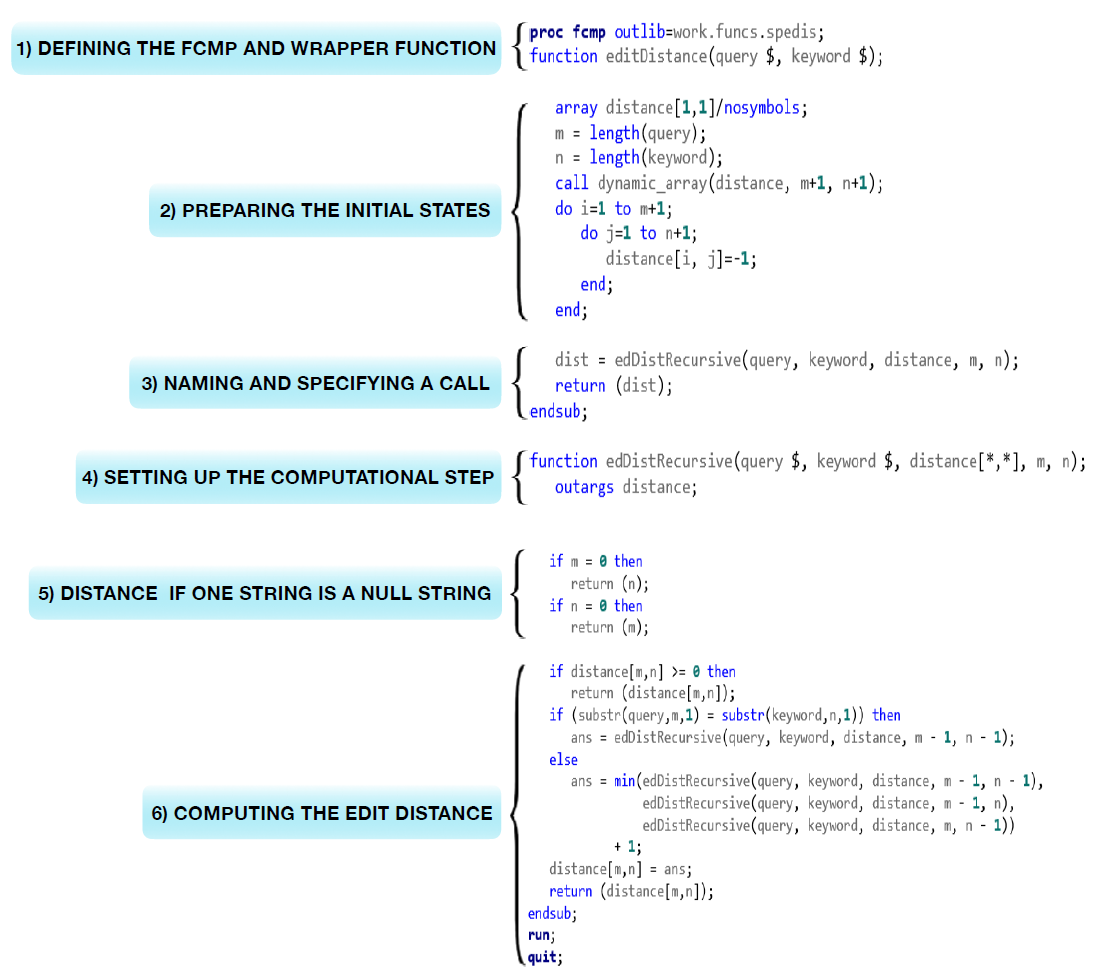Dynamic programming is a powerful technique to implement algorithms, and is often used to solve complex computational problems. Some are applications are world-changing, such as aligning DNA sequences; others are more "everyday," such as spelling correction. If you search for "dynamic programming," you will find lots of materials including sample programs written in other programming languages, such as Java, C, and Python etc., but there isn't any SAS sample program. SAS is a powerful language, and of course SAS can do it! This article will show you how to write your dynamic programming function with SAS FCMP through an example function used to calculate minimum edit distance between two strings.

Note that SAS offers built-in functions for edit distance, including the SPEDIS function. The purpose of this article is to demonstrate how you can implement such a function in a SAS dynamic programming method.

### What is edit distance?

Edit distance is a metric used to measure dissimilarity between two strings, by matching one string to the other through insertions, deletions, or substitutions.

### What is minimum edit distance?

Minimum edit distance measures the dissimilarity between two strings through the least number of edit operations.
Taking two strings "BAD" and "BED" as an example. These two words have multiple match possibilities. One solution is an edit distance of 1, resulting from one substitution from letter "A" to "E".Another solution might be deleting letter "A" from "BAD" then inserting letter "E" between "B" and "D", which results the edit distance of 2

There are several variants of edit distance, depending on the cost of edit operation. For example, given a string pair "BAD" and "BED", if each operation has cost of 1, then its edit distance is 1; if we set substitution cost as 2 (Levenshtein edit distance), then its edit distance is 2.

### What is dynamic programming?

The standard method used to solve minimum edit distance uses a dynamic programming algorithm.

Dynamic programming is a method used to resolve complex problems by breaking it into simpler sub-problems and solving these recursively. Partial solutions are saved in a big table, so it can be quickly accessed for successive calculations while avoiding repetitive work. Through this process of building on each preceding result, we eventually solve the original, challenging problem efficiently. Many difficult issues can be resolved using this method.

Here's the algorithm that solves Levenshtein edit distance through dynamic programming:The following image shows the annotated SAS program that implements the algorithm. The complete code (which you can copy and test for yourself) is at the end of this article.To demonstrate the edit distance function usage and validate the intermediate edit distance matrix table, I used a string pair "INTENTION" and "EXECUTION" that I copied from Stanford's class material as example. (This same resource also shows how the technique applies to DNA sequence alignment.)

```options cmplib=work.funcs; data test; infile cards missover; input word1 : \$20. word2 : \$20.; d=editDistance(word1,word2); put d=; cards; INTENTION EXECUTION ; run;```

The Levenshtein edit distance between "INTENTION" and "EXECUTION" is 8.

The edit distance table as follows.

 N 8 9 10 11 12 11 10 9 8 O 7 8 9 10 11 10 9 8 9 I 6 7 8 9 10 9 8 9 10 T 5 6 7 8 9 8 9 10 11 N 4 5 6 7 8 9 10 11 10 E 3 4 5 6 7 8 9 10 9 T 4 5 6 7 8 7 8 9 8 N 3 4 5 6 7 8 7 8 7 I 2 3 4 5 6 7 6 7 8 E X E C U T I O N

### More dynamic programming applications

Dynamic programming is a powerful technique, and it can be used to solve many complex computation problems. Anna Di and I are presenting a paper to the PharmaSUG 2018 China conference to demonstrate how to align DNA sequences with SAS FCMP and SAS Viya. If you are interested in this topic, please look for our paper after the conference proceedings are published.

### Appendix: Complete SAS program for editDistance function

```proc fcmp outlib=work.funcs.spedis; function editDistance(query \$, keyword \$); array distance[1,1]/nosymbols; m = length(query); n = length(keyword); call dynamic_array(distance, m+1, n+1); do i=1 to m+1; do j=1 to n+1; distance[i, j]=-1; end; end;   i_max=m; j_max=n;   dist = edDistRecursive(query, keyword, distance, m, n);   do i=i_max to 1 by -1; do j=1 to j_max; put distance[i, j] best3. @; end; put; end;   return (dist); endsub;   function edDistRecursive(query \$, keyword \$, distance[*,*], m, n); outargs distance;   if m = 0 then return (n); if n = 0 then return (m);   if distance[m,n] >= 0 then return (distance[m,n]); if (substr(query,m,1) = substr(keyword,n,1)) then delta = 0; else delta = 2;   ans = min(edDistRecursive(query, keyword, distance, m - 1, n - 1) + delta, edDistRecursive(query, keyword, distance, m - 1, n) + 1, edDistRecursive(query, keyword, distance, m, n - 1) + 1); distance[m,n] = ans; return (distance[m,n]); endsub; run; quit;   options cmplib=work.funcs; data test; infile cards missover; input word1 : \$20. word2 : \$20.; d=editDistance(word1,word2); put d=; cards; INTENTION EXECUTION ; run;```
Share